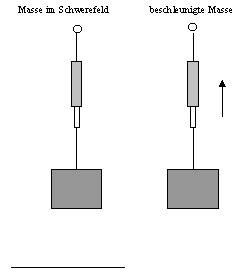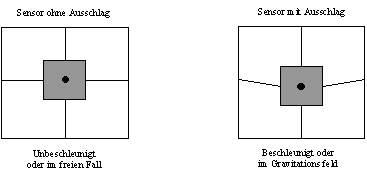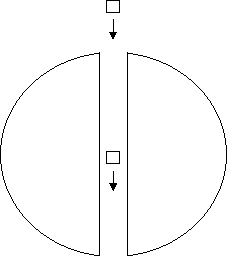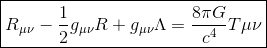# What is the basis of spacetime and it is infinite

### Introduction:

The general theory of relativity is also referred to as Einstein's theory of gravitation, since it replaces the concept of the gravitational field, which follows from Newton's theory, with the curvature of space-time. According to Newton's theory, two masses exert a force on each other, which leads to the masses moving towards each other with increasing speed, if they are not prevented from doing so by other forces (action at a distance).

Furthermore, the special theory of relativity only deals with reference systems that move relative to each other with constant speed. The generalization to systems accelerated relative to one another takes place in the general theory of relativity.

### Equivalence principle:

The fundamental idea that leads to the general theory of relativity is the equivalent way of looking at acceleration and gravity. (Equivalence principle)

In concrete terms, this means that in a closed space you cannot distinguish whether you are in a "gravitational field" or whether this space is accelerated accordingly.

As an example, a spring balance is shown here, which shows the same value in a gravitational field as with a corresponding acceleration.

The basic idea is now to treat acceleration and gravity in the same way, since these cannot be differentiated in a closed space in terms of measurement technology. This means that two phenomena that are treated differently in classical physics are combined into a single principle.### The free fall and the curvature of space-time

On the other hand, if you are in a closed space that is moving in free fall towards a planet without an atmosphere, there is no way within the space to measure the acceleration relative to the position of the planet.Sensor for measuring accelerations or gravitational fields

In the two drawings in the picture above, a sensor for measuring accelerations or an existing gravitational field is shown. The sensor consists of a mass that is suspended from four spring trolleys. (or six if you want to measure accelerations in all three dimensions of space.)

The first picture shows a sensor that is not accelerated or that moves in free fall in a gravitational field. In this case, the four spring balances do not show any deflection.

The second picture shows the same sensor when it is accelerated or the sensor does not move in a gravitational field. For example, the sensor could stand on a laboratory bench on the ground.

According to classical physics, the sensor is not in motion relative to the table. According to the general theory of relativity, however, the sensor on the table measures an acceleration.

In the following picture a very special planet is shown, with the help of which I would like to show how the movement of two bodies relative to each other, within a gravitational field, can be reinterpreted according to the general theory of relativity.

The planet should not have an atmosphere, because the frictional resistance of the atmosphere slows down the movement of a body and thus represents an acceleration. Furthermore, the planet should not rotate. A hole is drilled through the planet that is so large that two test specimens, both of which are equipped with a sensor for measuring the acceleration, can fall through this planet without any problems.Two inertial systems in the "gravitational field"

The two test specimens come from an area in space that is very far from our planet. The gravity of our planet is negligibly small at this distance. The two test baskets are now moving at the same distance in the direction of our planet.

The first specimen is accelerated more than the second specimen when they move in the direction of the planet. The distance between the two test specimens therefore increases. When the first specimen is in the center of our planet, it has the maximum speed and the maximum distance. Then the first specimen is slowed down again. When the two test specimens have again reached a large distance from the planet, they fly again at the same speed and the same distance relative to one another as at the beginning of the experiment.

During the entire traverse of the planet, the two test specimens with their built-in sensors did not measure any acceleration. An observer within these specimens would float. However, the two test specimens have the option of measuring the distance relative to one another by exchanging light pulses. Let us further assume that the hole in the planet is very large and that the planet could not be seen from the specimens. From the test specimens, therefore, it could not be determined that they were in a gravitational field. Acceleration was not measured in either of the two test specimens. The distance between the two bodies had changed for the time the planet was traversed, so space in this area of ​​the universe is curved.

We now want to consider a small, delimited space, e.g. a small cube with the name dVwhich has the following properties:

• The cube dV move freely in a gravitational field. So the cube is in free fall. A satellite orbiting the earth can be seen as a special form of free fall.
• The cube dV be chosen so small that the inhomogeneities of the gravitational field can be neglected.
• With the dice dV Let a coordinate system be firmly connected, which therefore moves with the cube.

If these requirements are met, the cube represents an inertial system. The laws of special relativity apply within this system.

ds2 = c2German2 - (dx2 + dy2 + dz2)

ds is the distance between two events inside the cube dV. The distances dt, dx, dy, dz lie inside the cube.

So within a gravitational field we have defined small spatial elements within which the special theory of relativity can be applied. With this we have a generalization of the special theory of relativity to a space with a gravitational field. Within such a generalized theory of relativity, the special theory of relativity only applies locally. The transformation between different local inertial systems provides information about the structure of space-time.

### Einstein's field equation

Einstein's field equation is the fundamental equation of general relativity.

This equation can be described as follows:
The mass defines the curvature of spacetime and the curved spacetime determines the movement of the mass.The Einstein field equation consists of the following elements:

• R.μν is the Ricci curvature tensor
• R. is the curvature scalar
• Λ is the cosmological constant
• G is the gravitational constant
• Gμν is the metric tensor
• c is the speed of light
• Tμν is the energy-momentum tensor

Einstein's field equation is a tensor equation and therefore consists of several individual equations. An equation corresponds to each element of the tensor. The individual elements of the tensor and thus the individual equations are based on the indices μ and ν Are defined. Both indices can have the values ​​from 0 to 3. These four values ​​represent the four dimensions of space-time. The value 0 corresponds to the time and 1, 2, 3 to the x, y, z Axes of space. Of the 16 possible variants, however, 6 equations are duplicates. A total of 10 equations are therefore available for calculations.

The left side of the equation describes the curvature of spacetime. The right side of the equation has to do with the distribution of mass and energy.